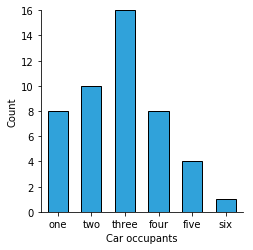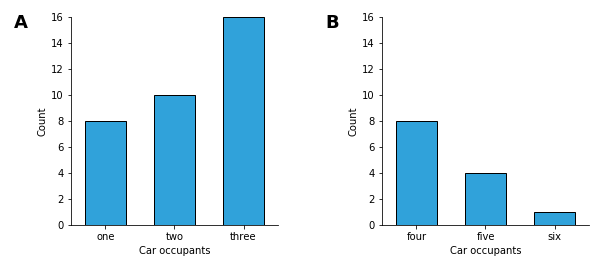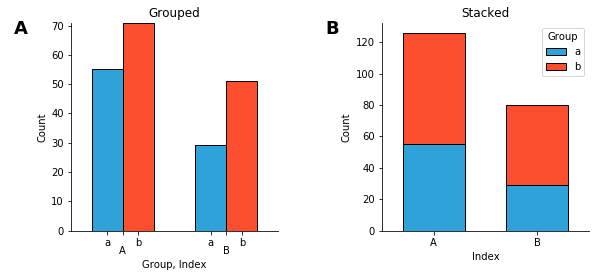# Bars¶

Title
Bars Element
Dependencies
Matplotlib
Backends
Matplotlib
Bokeh
In :
import numpy as np
import holoviews as hv
hv.extension('matplotlib')The Bars Element uses bars to show discrete, numerical comparisons across categories. One axis of the chart shows the specific categories being compared and the other axis represents a continuous value.

Bars may also be stacked by supplying a second key dimensions representing sub-categories. Therefore the Bars Element expects a tabular data format with one or two key dimensions and one value dimension. See the Tabular Datasets user guide for supported data formats, which include arrays, pandas dataframes and dictionaries of arrays.

In :
data = [('one',8),('two', 10), ('three', 16), ('four', 8), ('five', 4), ('six', 1)]
bars = hv.Bars(data, hv.Dimension('Car occupants'), 'Count')
bars

Out:You can 'slice' a Bars element by selecting categories as follows:

In :
bars[['one', 'two', 'three']] + bars[['four', 'five', 'six']]

Out:Bars support stacking just like the Area element as well as grouping by a second key dimension. When declaring a second key dimension Bars will visualize it as groupd bars by default to activate stacking instead set the stacked=True option:

In :
from itertools import product
np.random.seed(3)
index, groups = ['A', 'B'], ['a', 'b']
keys = product(index, groups)
bars = hv.Bars([k+(np.random.rand()*100.,) for k in keys],
['Index', 'Group'], 'Count')

grouped = bars.relabel('Grouped')
stacked = bars.relabel('Stacked')

grouped + stacked.opts(stacked=True)

Out:For full documentation and the available style and plot options, use hv.help(hv.Bars).